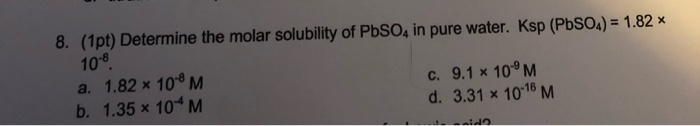# Which of the following compounds will have the highest molar solubility in pure water? PbSO4, Ksp = 1.82

Which of the following compounds will have the highest molar solubility in pure water?

1. PbSO4, Ksp = 1.82

so sulubility will be higher for that salt which will have higher ksp

and MgCO3 has higher ksp among the all of the above options

1)Ksp of PbSO4 = (solubility)^2

or, solubility = 1.35*10^-4 M

2) Ksp of MgCO3 = (solubility)^2

or, solubility = 2.612*10^-3 M

3) Ksp of AgI = (solubility)^2

or, solubility = 9.225*10^-9 M

4) Ksp of PbS = (solubility)^2

or, solubility = 9.51*10^-15 M

5) Ksp of FeS = (solubility)^2

or, solubility = 6.12*10^-10 M

thus, MgCO3 has the highest solubility

##### Add Answer of: Which of the following compounds will have the highest molar solubility in pure water? PbSO4, Ksp = 1.82
Similar Homework Help Questions
• ### Determine the molar solubility of PbSO4 in pure water. Ksp(PbSO4)= 1.82 x 10^-8.

Determine the molar solubility of PbSO4 in pure water. Ksp(PbSO4)= 1.82 x 10^-8.

• ### Determine the molar solubility of PbSO4 in pure water. Ksp (PbSO4) = 1.82 times 10-8.

Determine the molar solubility of PbSO4 in pure water. Ksp (PbSO4) = 1.82 times 10-8.

• ### 8. (1pt) Determine the molar solubility of PbSO, in pure water. Ksp (PbSO4) = 1.82 * 10-8 a. 1.82 * 10M C. 9.1 x 10...8. (1pt) Determine the molar solubility of PbSO, in pure water. Ksp (PbSO4) = 1.82 * 10-8 a. 1.82 * 10M C. 9.1 x 10°M b. 1.35 * 10*M d. 3.31 x 10-18 M

• ### Use the Ksp values to calculate the molar solubility of each of the following compounds in pure...

Use the Ksp values to calculate the molar solubility of each of the following compounds in pure water.Part B: PbCl2 (Ksp = 1.17×10-5)FYI-Answer is not 1.43*10^-2 M

• ### Use the Ksp values to calculate the molar solubility of each of the following compounds in pure...

Use the Ksp values to calculate the molar solubility of each of the following compounds in pure water.Part A: BaSO4 (Ksp = 1.07×10-10)Part B: PbCl2 (Ksp = 1.17×10-5)Part C: Ca(OH)2 ( Ksp = 4.68×10-6)

• ### Determine the molar solubility of PbSO4 in pure water. Ksp (PbSO4) = 1.6 × 10-10. a) 4.12 × 10-5 M b) 1.35 × 10-4 M c) 4...

Determine the molar solubility of PbSO4 in pure water. Ksp (PbSO4) = 1.6 × 10-10. a) 4.12 × 10-5 M b) 1.35 × 10-4 M c) 4.89 × 10-4 M d) 1.26 × 10-5 M e) 1.28 × 10-8 M

• ### Determine the molar solubility of MgCO3 in pure water. Ksp (MgCO3) = 6.82

Determine the molar solubility of MgCO3 in pure water. Ksp (MgCO3) = 6.82

• ### Use the Ksp values to calculate the molar solubility of each of the following compounds in...

Use the Ksp values to calculate the molar solubility of each of the following compounds in pure water. Part A MX ( K sp = 5.30×10−11) Express your answer in moles per liter. Part B Ag2CrO4 (Ksp = 1.12×10−12) Express your answer in moles per liter. Part C Ni(OH)2 (Ksp = 5.48×10−16) Express your answer in moles per liter.

• ### The molar solubility of ZnS is 1.6x10-12 M in pure water. Calculate the Ksp for ZnS.

The molar solubility of ZnS is 1.6x10-12 M in pure water. Calculate the Ksp for ZnS.

• ### What is the molar solubility of calcium sulfate in pure water?

Ksp=2.4x10-5 for calcium sulfate. A. What is the molar solubility of calcium sulfate in pure water? B. What is the mass solubility of calcium sulfate in pure water, expressed in g/L?

Need Online Homework Help?